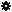﻿ Object Model: PolygonFace.GrowNeighborPolygons

PolygonFace.GrowNeighborPolygons

## PolygonFace.GrowNeighborPolygonsv3.0

### Description

Returns a collection of PolygonFace objects for all polygons that are adjacent to each Vertex in this PolygonFace, within a given distance.

### C# Syntax

 ```PolygonFaceCollection PolygonFace.GrowNeighborPolygons( Int32 in_lDistance ); ```

### Scripting Syntax

 ```oReturn = PolygonFace.GrowNeighborPolygons( [Distance] ); ```

### Parameters

Parameter Type Description
Distance Integer Distance is an integer value representing the degree of neighborhood (eg: degree=2 for a polygon means its adjacent polygons plus the adjacent polygons of the adjacent polygons)

Default Value: 1

### Examples

#### VBScript Example

 ```set oCube = ActiveSceneRoot.AddGeometry( "Cone", "MeshSurface" ) set oGeometry = oCube.ActivePrimitive.Geometry set oPolygons = oGeometry.Polygons(0) set oNeighborPolygons = oPolygons.GrowNeighborPolygons str = "Polygons around the polygon(0) are :" for each n in oNeighborPolygons str = str & " " & n.index next logmessage str ```